﻿ SQL Server LEFT() and RIGHT() functions

# SQL Server LEFT() and RIGHT() functions

SQL Server built-in functions provide many useful functions to perform various operations on data. The data can range from String, Texts or Numeric. This article highlights the use of LEFT() and RIGHT() functions from the lists of built-in functions.

Both LEFT() and RIGHT() functions take two arguments each and return values accordingly.

## Using SQL Server LEFT() function

This function will extract characters from the left part of a given string (text) value. The given value can either be a String or a Column of a table.

### Syntax

LEFT (string_value, number_of_characters)

The first argument can be a string inside the single quotes (' ') or it can be a value extracted from a given column name in a table. The second argument will be a numeric value which specifies the number of character(s) the user wishes to be returned by the query. We will see examples using both the cases.

## SQL Server LEFT() function with a “String”

```DECLARE @String VARCHAR (30)
SET @String = 'I AM A PROGRAMMER'

SELECT @String AS ActualValue, LEFT(@String, 10) AS Using_LEFT
```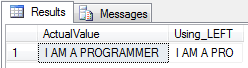## SQL Server LEFT() function using a table “Column”

For the demo we are using dbo.Books table which we have already created and you can find it here.

The table has a column named Category and we will use the function to get 3 characters from the left part of each categories. We can use the retuned values as short names.

```SELECT Category, LEFT(Category, 3) Shortend_Using_LEFT FROM dbo.Books
```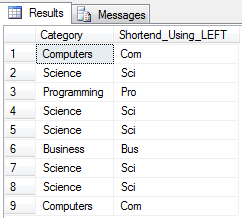## Using the RIGHT() function

It will extract characters or data from the right part of a given string value.

RIGHT (string_value, number_of_characters)

## RIGHT() function with a “String”

```DECLARE @String VARCHAR (30)
SET @String = 'I LIKE SQL SERVER'

SELECT @String AS ActualValue, RIGHT(@String, 10) AS Using_RIGHT
```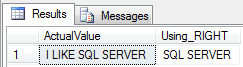## SQL Server RIGHT() function using a table “Column”

The dbo.Books table has another column named price and we will find the decimal values of each price listed in the table.

```SELECT Price, RIGHT(Price, 3) Using_RIGHT FROM dbo.Books
```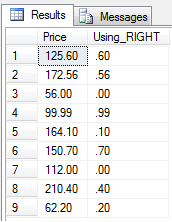## Using SQL Server LEFT() and SQL Server RIGHT() function together

Finally we will see the combine usage of both the functions in a single query and find out how useful it can be. Let us assume we have thousands of books in our inventory and we want a list of books and its prices which fall in the price range of 80 (can be any currency).

We will use the Price_Range column for this purpose. Price_Range simply denotes what range the books prices fall into.

```SELECT *FROM dbo.Books WHERE CAST(80 AS NUMERIC(18,2)) BETWEEN
LEFT(Price_Range, CHARINDEX('-',Price_Range) - 1) AND
RIGHT(Price_Range, LEN(Price_Range) - CHARINDEX('-',Price_Range))
```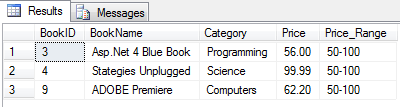In the above query, we have used another Sql built-in function CHARINDEX() to determine the exact location of the hyphen. We need to eliminate the hyphens in our query to make the figures comparable.

The CHARINDEX() function returns an integer value, which is what the LEFT() and RIGHT() function needs to get the desired result. Once we get the values from the range, we will compare our price using the BETWEEN operator which is ideal for selecting values in a range.

This ain't so complicated, but this surely will help us understand some of the basic functions in SQL Server. These little functions when used in sync with a large query can deliver great results.